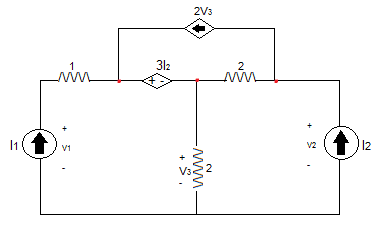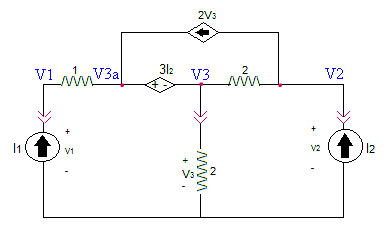# Nodal analysis

magnifik
For the following circuit, can someone please correct my equations for nodal analysis (KCL)I am especially unsure about my second equation because of the dependent voltage source to the left of the node.

1) I1 = (V1 - 3I2)/1
2) 0 = V3/2 + (V3 -V2)/2
3) I2 = (V2-V3)/2 + 2V3

Staff Emeritus
(1) and (2) are wrong.

N.B., current flows though every element in the circuit.

magnifik
(1) and (2) are wrong.

N.B., current flows though every element in the circuit.

the dependent sources are throwing me off. are the equations at least partially correct?

Mentor
the dependent sources are throwing me off. are the equations at least partially correct?

Your third equation is okay. The first equation is ignoring the fact that the controlled voltage source 3I2 is connected to V3, not ground. The second equation is ignoring any current that may be flowing into the V3 node via the 3I2 source.

What is it you are expected to solve for? Is it the three voltages in terms of currents I1 and I2?

You might find it beneficial to write an equation in terms of the supernode (as indicated by the line breaks):#### Attachments

magnifik
Your third equation is okay. The first equation is ignoring the fact that the controlled voltage source 3I2 is connected to V3, not ground. The second equation is ignoring any current that may be flowing into the V3 node via the 3I2 source.

What is it you are expected to solve for? Is it the three voltages in terms of currents I1 and I2?

ultimately, i will be solving for y-param. i was able to do so using mesh analysis instead, but i was hoping to try and obtain the nodal equations to double check my answers.

Gold Member
ultimately, i will be solving for y-param. i was able to do so using mesh analysis instead, but i was hoping to try and obtain the nodal equations to double check my answers.

Are you wanting the Y parameters only for the two-port? That is, just for V1-I1 as the input and V2-I2 as the output, in effect reducing the whole circuit to just a two-port?